# A 400-lb vertical force is applied at D to a gear attached to the solid 1-in. diameter shaft AB. Determine the principal stresses and the maximum shearing stress at point H located as shown on top of the shaft.

Question-AnswerCategory: Strength of MaterialsA 400-lb vertical force is applied at D to a gear attached to the solid 1-in. diameter shaft AB. Determine the principal stresses and the maximum shearing stress at point H located as shown on top of the shaft.

A 400-lb vertical force is applied at D to a gear attached to the solid 1-in. diameter shaft AB. Determine the principal stresses and the maximum shearing stress at point H located as shown on top of the shaft.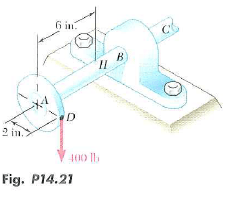Step No: 1

Consider a section at H.
Calculate the moment at H using the formula,
Moment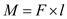Here, F is the force and l is the perpendicular distance of the line of action of force from H.
Substitutefor F and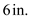for l.Step No: 2

Calculate the torque at H using the formula,
TorqueHere,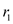is the radius of the gear.
Substitute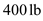for F andfor.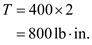Step No: 3

Calculate the polar moment of inertia of the shaft using the formula,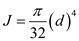Here, d is the diameter of the shaft.
Substitute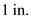for d.Calculate the moment of inertia of the shaft using the relation,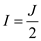Substitute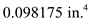for J.Step No: 4

Calculate the shear stress using the relation,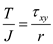Here, r is the radius of the shaft.
Substituteforfor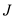, and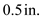for r.Step No: 5

Calculate the bending stress using the relation,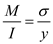Here, y is the half of the diameter of the shaft.
Substitutefor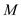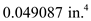for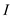, andfor y.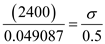Step No: 6

Transverse shear stress at point H is zero.
Write the resultant stress.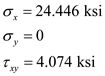Calculate the average stress as follows: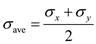Substitutefor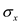and 0 for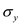.Step No: 7

Calculate the maximum shear stress as follows: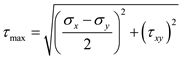Substitutefor, 0 for, andfor.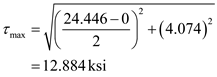Therefore the maximum shear stress is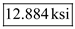.

Step No: 8

Calculate the maximum principal stress using the formula,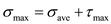Substitute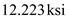for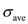and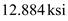for.Therefore, the maximum principal stress is.

Step No: 9

Calculate the minimum principal stress using the formula,Substituteforandfor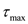.Therefore, the minimum principal stress is.